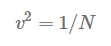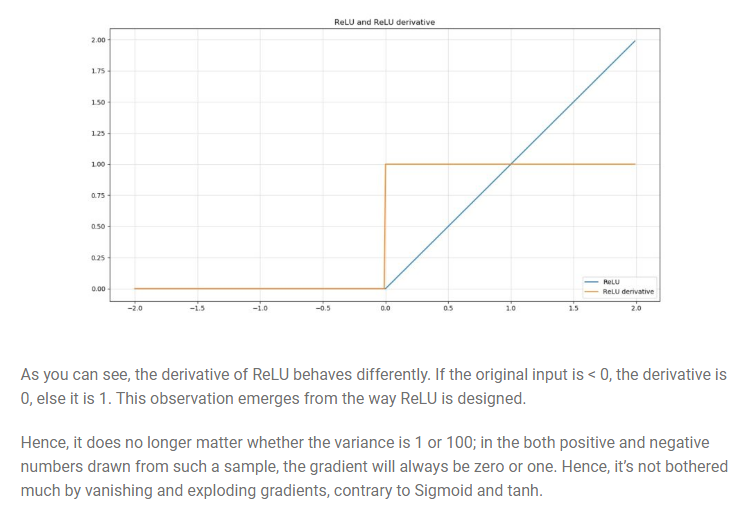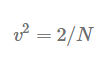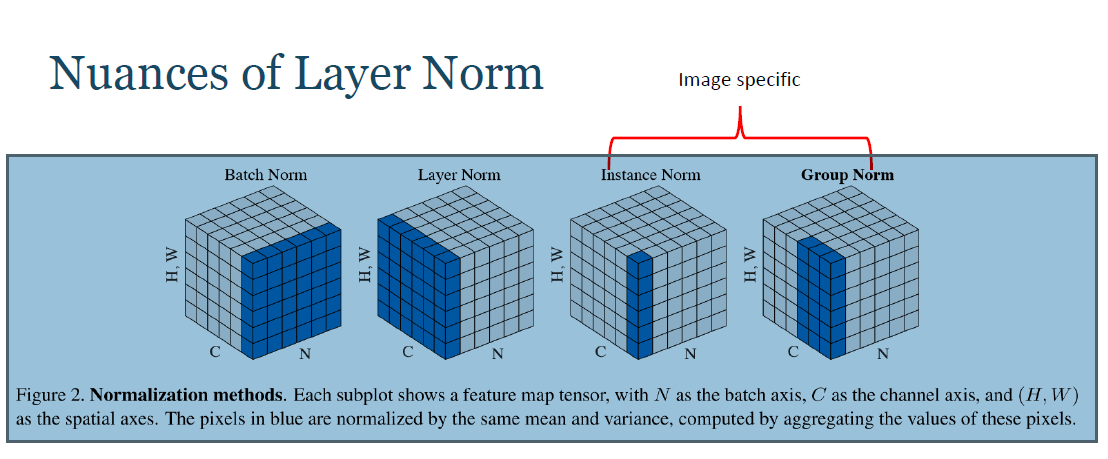1596826560

# Weights Initialization

## The importance of effective initialization

To build a machine learning algorithm, usually you’d define an architecture (e.g. Logistic regression, Support Vector Machine, Neural Network) and train it to learn parameters. Here is a common training process for neural networks:

1. Initialize the parameters
2. Choose an optimization algorithm
3. Repeat these steps:
4. Forward propagate an input
5. Compute the cost function
6. Compute the gradients of the cost with respect to parameters using backpropagation
7. Update each parameter using the gradients, according to the optimization algorithm

Then, given a new data point, you can use the model to predict its class.

The initialization step can be critical to the model’s ultimate performance, and it requires the right method. To illustrate this, consider the three-layer neural network below. You can try initializing this network with different methods and observe the impact on the learning.

Case 1: A too-large initialization leads to exploding gradients

If weights are initialized with very high values the term `np.dot(W,X)+b` becomes significantly higher and if an activation function like sigmoid() is applied, the function maps its value near to 1 where the slope of gradient changes slowly and learning takes a lot of time.When these activations are used in backward propagation, this leads to the exploding gradient problem. That is, the gradients of the cost with the respect to the parameters are too big. This leads the cost to oscillate around its minimum value.

Case 2: A too-small initialization leads to vanishing gradients

If weights are initialized with low values it gets mapped to 0.

When these activations are used in backward propagation, this leads to the vanishing gradient problem. The gradients of the cost with respect to the parameters are too small, leading to convergence of the cost before it has reached the minimum value.

or **intuitively **we can use the below reasoning to understand the above mentioned points:

• When your weights and hence your gradients are close to zero, the gradients in your upstream layers vanish because you’re multiplying small values and e.g. 0.1 x 0.1 x 0.1 x 0.1 = 0.0001. Hence, it’s going to be difficult to find an optimum, since your upstream layers learn slowly.
• The opposite can also happen. When your weights and hence gradients are > 1, multiplications become really strong. 10 x 10 x 10 x 10 = 1000. The gradients may therefore also explode, causing number overflows in your upstream layers, rendering them “untrainable” (even dying off the neurons in those layers).

So thus we can conclude that we have to keep the variances of the weights initialized approximately equal to 1 across all layers.

# Xavier initialization

We need to pick the weights from a Gaussian distribution with zero mean and a variance of 1/N, where N specifies the number of input neurons.

With this strategy, which essentially assumes random initialization from e.g. the standard normal distribution but then with a specific variance that yields output variances of 1.This is for TanH function# He initializationWhen your neural network is ReLU activated, He initialization is one of the methods to chose, Mathematically it attempts to do the same thing

This difference is related to the nonlinearities of the ReLU activation function, which make it non-differentiable at x=0. However at other values it is either 0 or 1 as explained in the image above .The best weight initialization strategy is to initialize the weights randomly but with this variance:# Normalization methods:

Let us recall the meanings of Normalization and standardization in it’s most basic form.

A typical normalization process consists of scaling numerical data down to be on a scale from zero to one, and a typical standardization process consists of subtracting the mean of the dataset from each data point, and then dividing that difference by the data set’s standard deviation.

This forces the standardized data to take on a mean of zero and a standard deviation of one. In practice, this standardization process is often just referred to as normalization as well.

#artificial-neural-network #neural networks

## Buddha Community1596826560

# Weights Initialization

## The importance of effective initialization

To build a machine learning algorithm, usually you’d define an architecture (e.g. Logistic regression, Support Vector Machine, Neural Network) and train it to learn parameters. Here is a common training process for neural networks:

1. Initialize the parameters
2. Choose an optimization algorithm
3. Repeat these steps:
4. Forward propagate an input
5. Compute the cost function
6. Compute the gradients of the cost with respect to parameters using backpropagation
7. Update each parameter using the gradients, according to the optimization algorithm

Then, given a new data point, you can use the model to predict its class.

The initialization step can be critical to the model’s ultimate performance, and it requires the right method. To illustrate this, consider the three-layer neural network below. You can try initializing this network with different methods and observe the impact on the learning.

Case 1: A too-large initialization leads to exploding gradients

If weights are initialized with very high values the term `np.dot(W,X)+b` becomes significantly higher and if an activation function like sigmoid() is applied, the function maps its value near to 1 where the slope of gradient changes slowly and learning takes a lot of time.When these activations are used in backward propagation, this leads to the exploding gradient problem. That is, the gradients of the cost with the respect to the parameters are too big. This leads the cost to oscillate around its minimum value.

Case 2: A too-small initialization leads to vanishing gradients

If weights are initialized with low values it gets mapped to 0.

When these activations are used in backward propagation, this leads to the vanishing gradient problem. The gradients of the cost with respect to the parameters are too small, leading to convergence of the cost before it has reached the minimum value.

or **intuitively **we can use the below reasoning to understand the above mentioned points:

• When your weights and hence your gradients are close to zero, the gradients in your upstream layers vanish because you’re multiplying small values and e.g. 0.1 x 0.1 x 0.1 x 0.1 = 0.0001. Hence, it’s going to be difficult to find an optimum, since your upstream layers learn slowly.
• The opposite can also happen. When your weights and hence gradients are > 1, multiplications become really strong. 10 x 10 x 10 x 10 = 1000. The gradients may therefore also explode, causing number overflows in your upstream layers, rendering them “untrainable” (even dying off the neurons in those layers).

So thus we can conclude that we have to keep the variances of the weights initialized approximately equal to 1 across all layers.

# Xavier initialization

We need to pick the weights from a Gaussian distribution with zero mean and a variance of 1/N, where N specifies the number of input neurons.

With this strategy, which essentially assumes random initialization from e.g. the standard normal distribution but then with a specific variance that yields output variances of 1.This is for TanH function# He initializationWhen your neural network is ReLU activated, He initialization is one of the methods to chose, Mathematically it attempts to do the same thing

This difference is related to the nonlinearities of the ReLU activation function, which make it non-differentiable at x=0. However at other values it is either 0 or 1 as explained in the image above .The best weight initialization strategy is to initialize the weights randomly but with this variance:# Normalization methods:

Let us recall the meanings of Normalization and standardization in it’s most basic form.

A typical normalization process consists of scaling numerical data down to be on a scale from zero to one, and a typical standardization process consists of subtracting the mean of the dataset from each data point, and then dividing that difference by the data set’s standard deviation.

This forces the standardized data to take on a mean of zero and a standard deviation of one. In practice, this standardization process is often just referred to as normalization as well.

#artificial-neural-network #neural networks

1603278729

## All About Artificial Neural Networks – What, Why, and How?

If you are interested in Artificial Intelligence, chances are that you must have heard about Artificial Neural Networks (ANN), and Deep Neural Networks (DNN). This article is about ANN.

The boom in the field of artificial intelligence may have come recently, but the idea is old. The term AI was coined way back in 1956. Its revival though in the 21st century can be traced to 2012 when ImageNet challenge. Before this, AI was known as neural networks or expert systems.

At the foundation of AI are the networks of artificial neurons, the same as the cells of a biological brain. Just like every neuron can be triggered by other neurons in a brain, AI works similarly through ANNs. Let’s know more about them.

Artificial Neural Networks – Borrowing from human anatomy
Popularly known as ANN, Artificial Neural Network is basically a computational system, which is inspired by the structure, learning ability, and processing power of a human brain.

ANNs are made of multiple nodes imitating the neurons of the human brain. These neurons are connected by links and also interact with each other. These nodes facilitate the input of data. The structure in ANN is impacted by the flow of information, which changes the neural networks based on the input and output.

A simple, basic-level ANN is a “shallow” neural network that has typically only three layers of neurons, namely:

Input Layer. It accepts the inputs in the model.
Hidden Layer.
Output Layer. It generates predictions.

#artificial neural networks #neural networks #ai #ml #artificial intelligence1602874800

## Introduction

ANNs (Artificial Neural Network) is at the very core of Deep Learning an advanced version of Machine Learning techniques. ANNs are versatile, adaptive, and scalable, making them appropriate to tackle large datasets and highly complex Machine Learning tasks such as image classification (e.g., Google Images), speech recognition (e.g., Apple’s Siri), video recommendation (e.g., YouTube), or analyzing sentiments among customers (e.g. Twitter Sentiment Analyzer).

ANN was first introduced in 1943 by the neurophysiologist Warren McCulloch and the mathematician Walter Pitts. However, ANN had its ups and downs. Post-1960 there was a drop in interest and excitement among researchers w.r.t neural networks with the advancement of Support Vector Machines and other powerful Machine Learning techniques that produced better accuracy and had a stronger theoretical foundation. Neural networks were complex and required tremendous computation power and time to train. However post 1990, the advancement in the field of computation (refer to Moore’s law) followed by the production of powerful GPU cards brought some interest back.

#data-science #neural-networks #machine-learning #artificial-neural-network #artificial-intelligence1598313600

## Introduction to Neural Networks

There has been hype about artificial intelligence, machine learning, and neural networks for quite a while now. I have been working on these things for over a year now so I would like to share some of my knowledge and give my point of view on Neural networks. This will not be a math-heavy introduction because I just want to build the idea here.

I will start from the neural network and then I will explain every component of a neural network. If you feel like something is not right or need any help with any of this, Feel free to contact me, I will be happy to help.

## When to use the Neural Network?

Let’s assume we want to solve a problem where you are given some set of images and you have to build an automated system that can categories each of those images to its correct label.

The problem looks simple but how do we come with some logic using raw pixel values and target labels. We can try comparing pixels and edges but we won’t be able to come with some idea which can do this task effectively or say the accuracy of 90% or more.

When we have this kind of problem where we have high dimensional data like Images and we don’t know the relationship between Input(Images) and the Output(Labels), In this kind of scenario we should use Neural Networks.ư

## What is the Neural network?

Artificial neural networks, usually simply called neural networks, are computing systems vaguely inspired by the biological neural networks that constitute animal brains. An ANN is based on a collection of connected units or nodes called artificial neurons, which loosely model the neurons in a biological brain

#artificial-intelligence #gradient-descent #artificial-neural-network #deep-learning #neural-networks #deep learning1602954000

## Perceptron to Multi-layered Feedforward Neural Network

This article will help you understand the transition of AI from classical machine learning to deep learning, starting from the basics of machine learning with its major - supervised and unsupervised learning to different regularization and optimization techniques.

The goal of this article is to introduce the major concepts of machine learning-what is machine learning? How does a machine learn by itself to do a particular task? How does it choose the essential features of the data that strongly contribute to the prediction of future events? How can we understand whether a machine has succeeded or failed in that?

In this article, we will discuss the foundations of artificial neural networks starting from perceptron to multi-layered feedforward neural networks. This article discusses the various stages of how to apply different transformation techniques for data preparation, how to train a neural network, and then validate and deploy a neural network for solving real-world problems.

In this article, we’re going to cover the following main topics:

· Understanding Machine Learning and Artificial Neural Network

· Feedforward Neural Network & Backpropagation Algorithm

· Evaluating and Tuning the Artificial Neural Network

· Classical Machine Learning vs Deep Learning

## Understanding Machine Learning and Artificial Neural Network

This section starts with a brief overview of what is machine learning, its major types- supervised and unsupervised learning. Then we will understand the very evolution of artificial neural networks starting with how a biological neuron works. We’ll also discuss the design of artificial neurons with an understanding of deep neural networks with activation functions.

### What is Machine Learning?

The term, Machine learning, has become a buzzword nowadays which refers to the ability of a machine to learn from the data without the help of the set of rules that are defined explicitly as like in the traditional rule-based algorithms. So definitely, if it learns from the data without any need for an explicit declaration of rules then it has to do with the experience from learning.

Our way of learning always follows a curve of failures although it’s perfectly descendent, lastly it will converge to the extent of our hard work

In the last decade of technology, machine learning techniques have become the common tools to automate the tasks that would have required huge efforts with the traditional rule-based algorithms.

In the** traditional rule-based algorithms**, the set of rules used to be defined to work on with a specific variety of data and could not be generalized to a large extent of data because of its specificity of working on only particular data. For example, if YouTube, a video sharing site decides to perform a copyright check on videos that are being uploaded on its server with a human operator, it will need a lot many people to execute this task of copyright check. But if YouTube chooses to do this with the help of some video processing algorithm then the task of copyright check would be easier but not robust as video processing algorithm possibly would work only on a set of videos that don’t have any kind of transformations like flip, rotate, crop, blur, etc. And it’s quite difficult to write a separate algorithm for individual transformation so the solution to this problem can be machine learning. In this case, a learning model is built by getting trained on data and identifying implicit features that uniquely signifies the data with which new data can be validated automatically.

Today, we are living in the era of machine-learning-based technologies; email services learn how to classify the emails into spam and ham; search engines learn what to recommend to the user based on their search history; banking systems are now able to sanction loans based on the creditworthiness of a customer. Prediction of heart disease based on clinical data, identifying voice commands, and forecasting annual rainfall are other significant tasks that machine learning facilitates.

One common problem with all of these applications is that a programmer cannot explicitly define the set of instructions for the task that needs to be performed due to the underlying complexity of the data; this was machine learning helps. It has made itself useful across industries like retail, banking, healthcare to the automobile industry for its ability to predict future events with significant accuracy.

_In machine learning algorithms, the __input is the experience in form of data _and output is knowledge or wisdom gained with inductive inference which in turn helps to predict future events, so rather, machine learning is an art of experiential learning.

Let us start with a real-life experience of preparing a food dish with some cooking recipe, how do we prepare the food, let’s go through the process of making the delicious food, at first we collect all the ingredients that are needed for food preparation, as a naïve person in the cooking, we follow a cooking recipe which involves set of steps that need to be performed. Let us take an example of a famous dish of western India, poha, which needs many ingredients like beaten rice flakes, mustard, curry leaves, groundnuts, oil, salt, and others. Assume now, with all ingredients, we start making the poha as per the directions in the cooking recipe.

#artificial-intelligence #machine-learning #artificial-neural-network #neural-networks #deep-learning